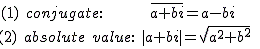# Complex Conjugate Calculation with Real and Imaginary numbers#Complex Conjugate,Absolute Calculator

An online simple and complex conjugate, Absolute calculation.

 +- iSimple Conjugate Function

Conjugate of X+Y is X-Y, where X and Y are real numbers

Complex Conjugate Function

Conjugate of X+Y is X-Y, where X and Y are real and an imaginary numbers

## Complex Conjugate & Absoulte Formula##Top Calculators ►

Online Algebra calculation, formulas , Digital calculation, Statistical calculation, Math Converters Pet Age Calculator,